# RS Aggarwal Solutions for Class 9 Chapter 15: Volume and Surface Area of Solids Exercise 15C

## RS Aggarwal Solutions for Class 9 Maths Exercise 15C PDF

Students can enhance their problem solving abilities using the RS Aggarwal Solutions prepared by our subject experts. These solutions contain answers to the problems which are based on the latest CBSE syllabus. The subject experts at BYJU’S create the solutions with the aim of helping students score well in the board exams. Practising the exercise wise solutions help students improve their confidence from exam point of view. Proper explanations and illustrations are provided in order to help students understand the concepts faster. RS Aggarwal Solutions for Class 9 Maths Chapter 15 Volume and Surface Area of Solids Exercise 15C are provided here.

## RS Aggarwal Solutions for Class 9 Chapter 15: Volume and Surface Area of Solids Exercise 15C Download PDF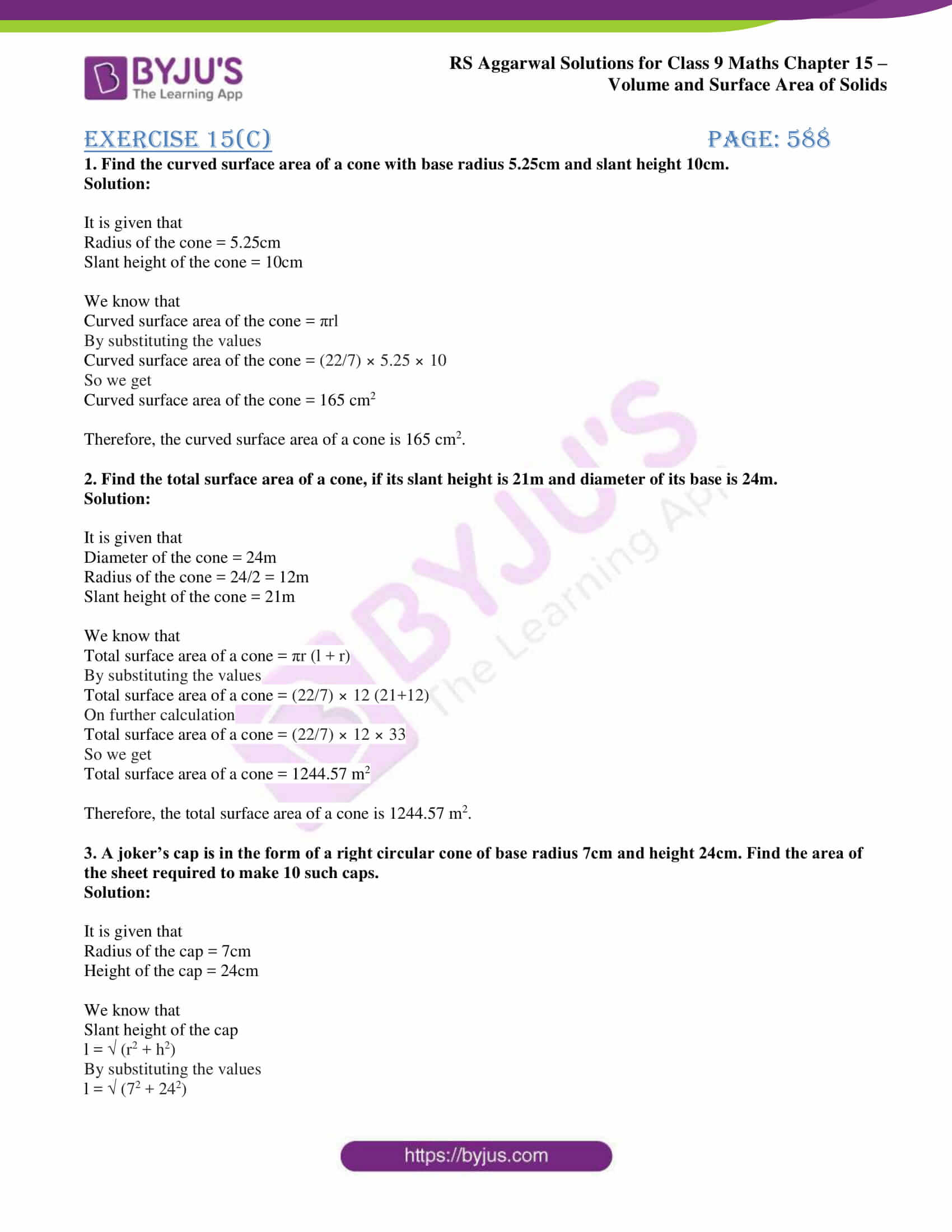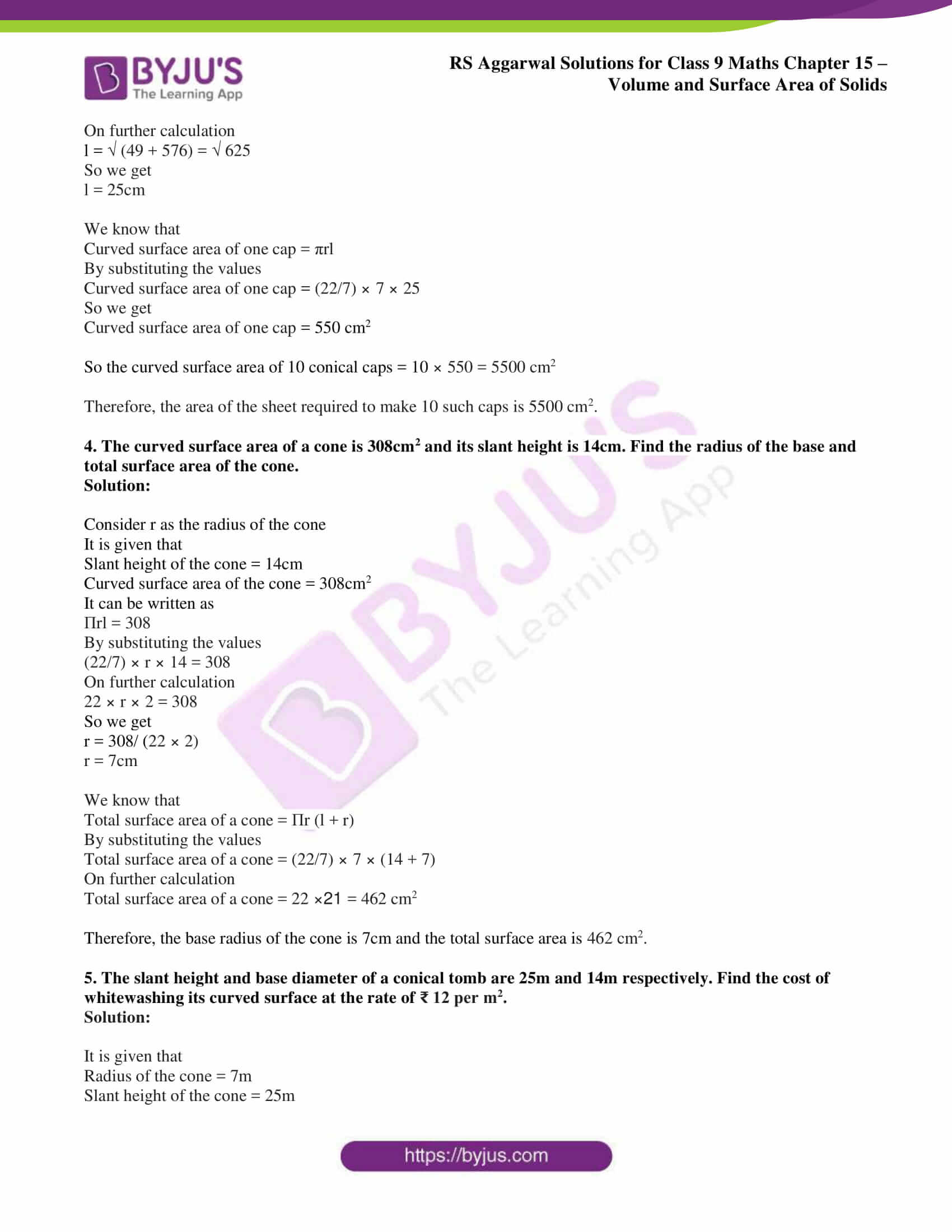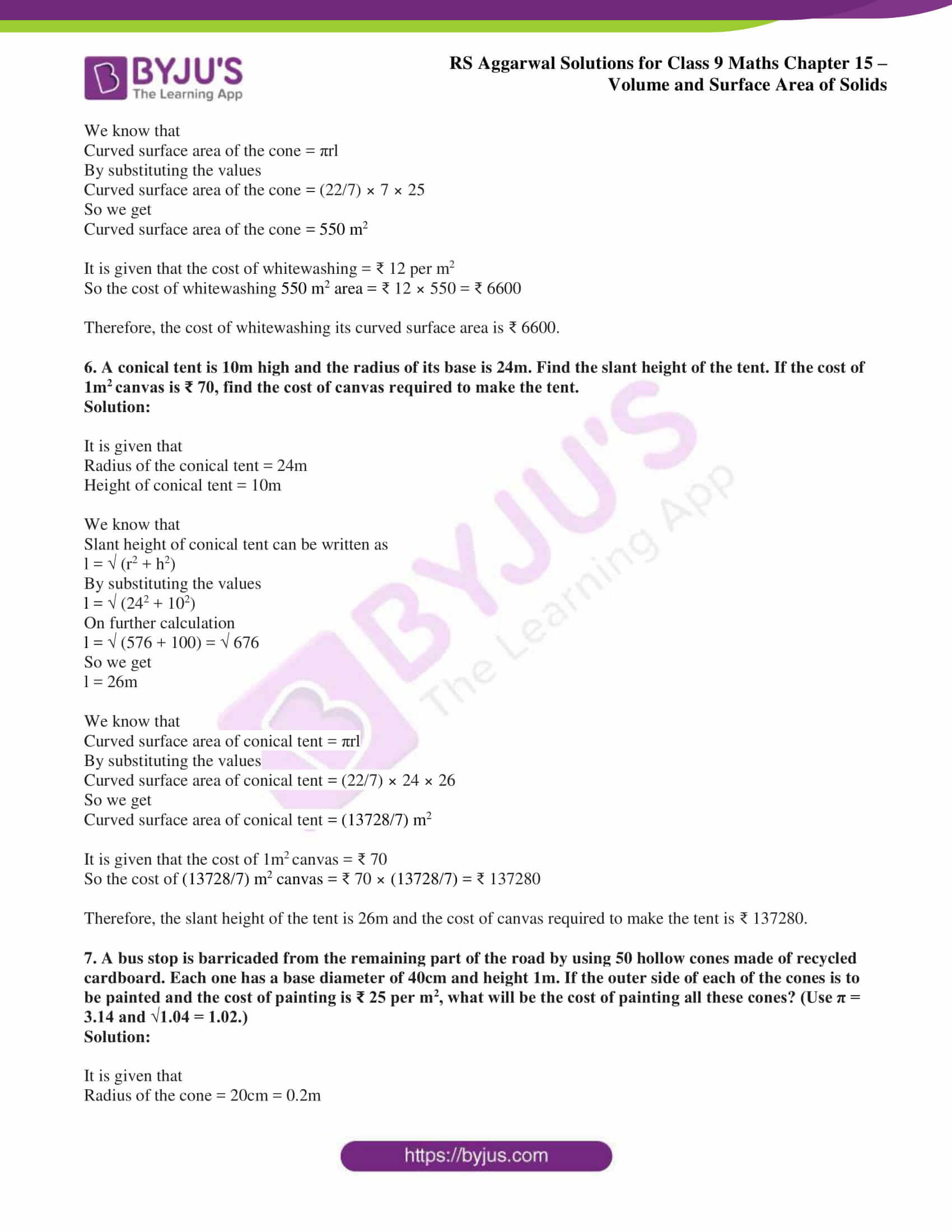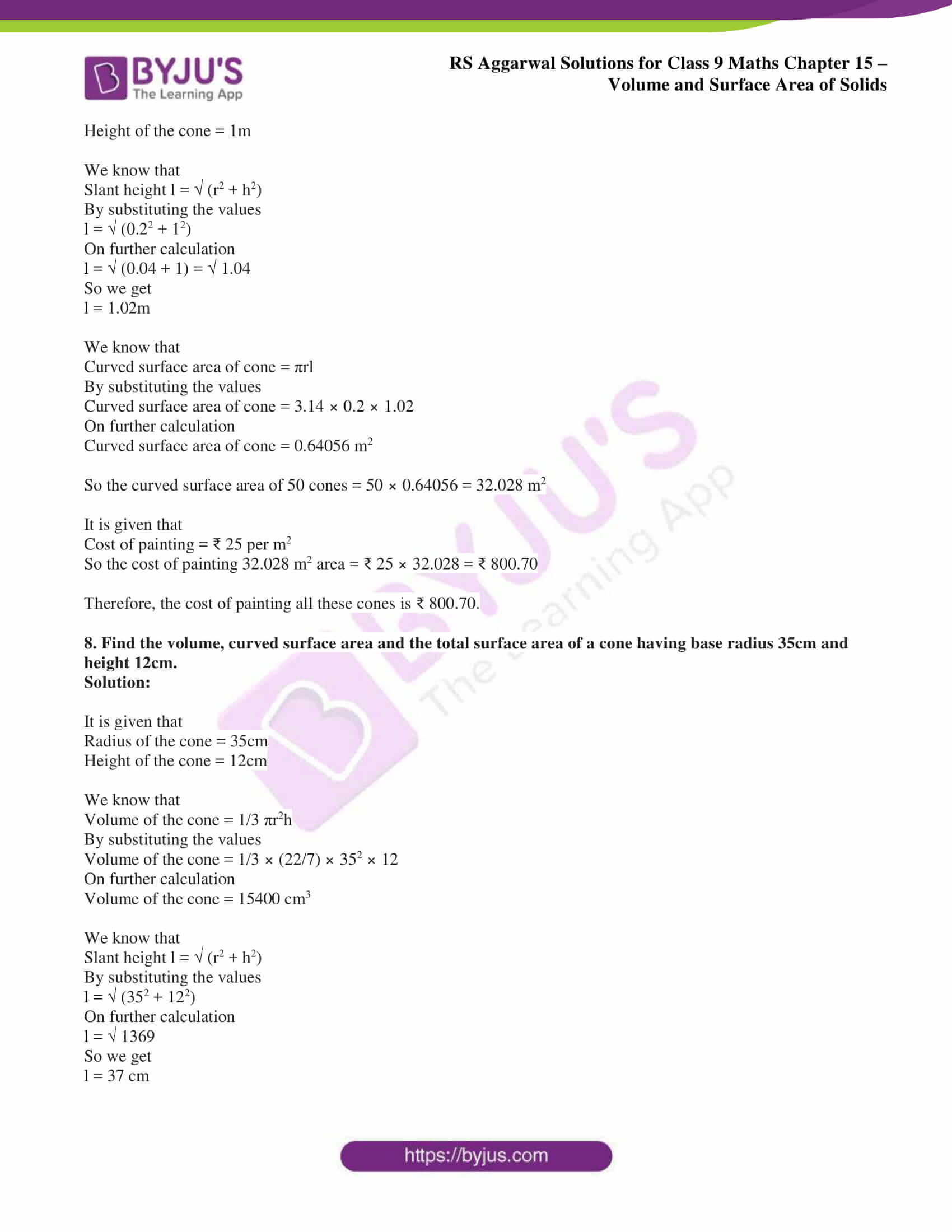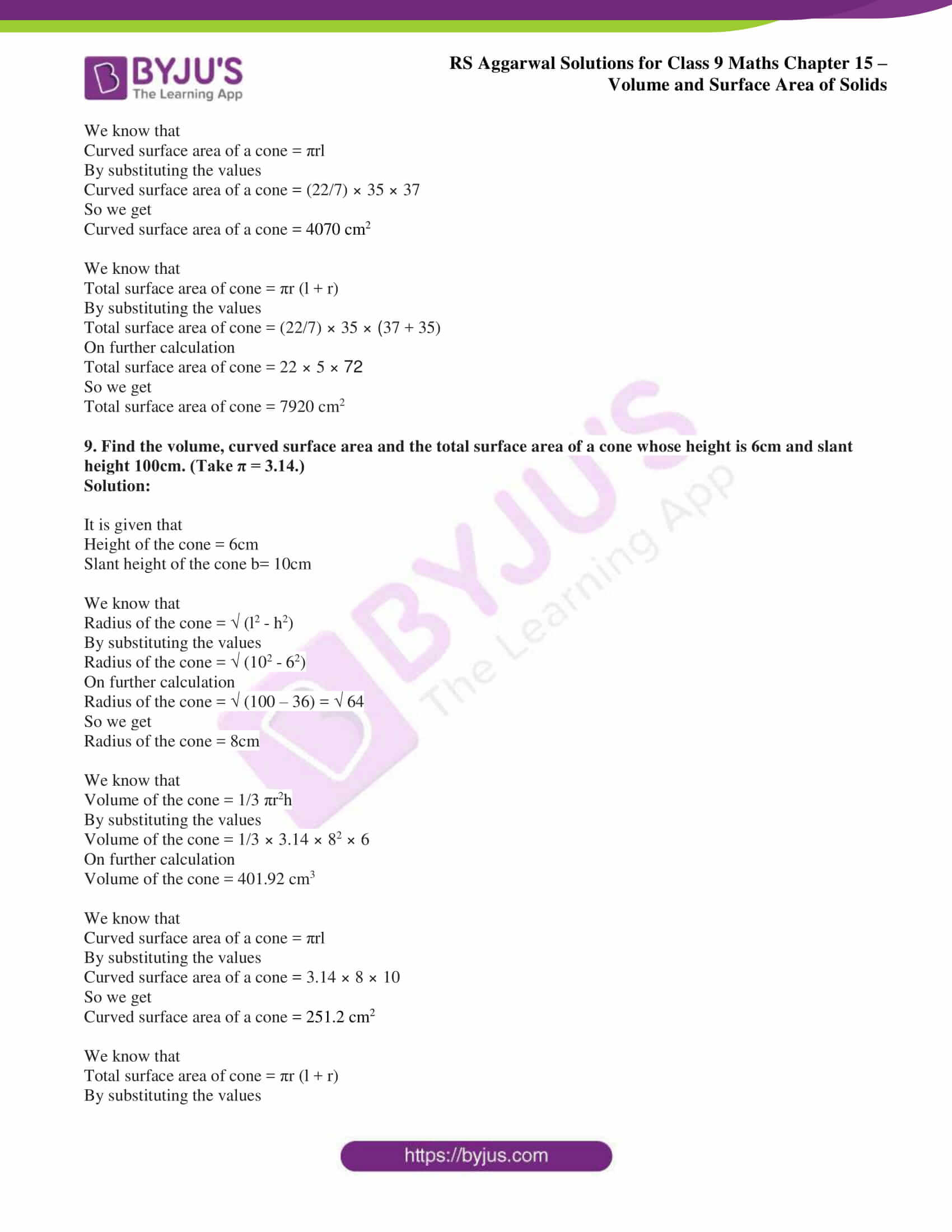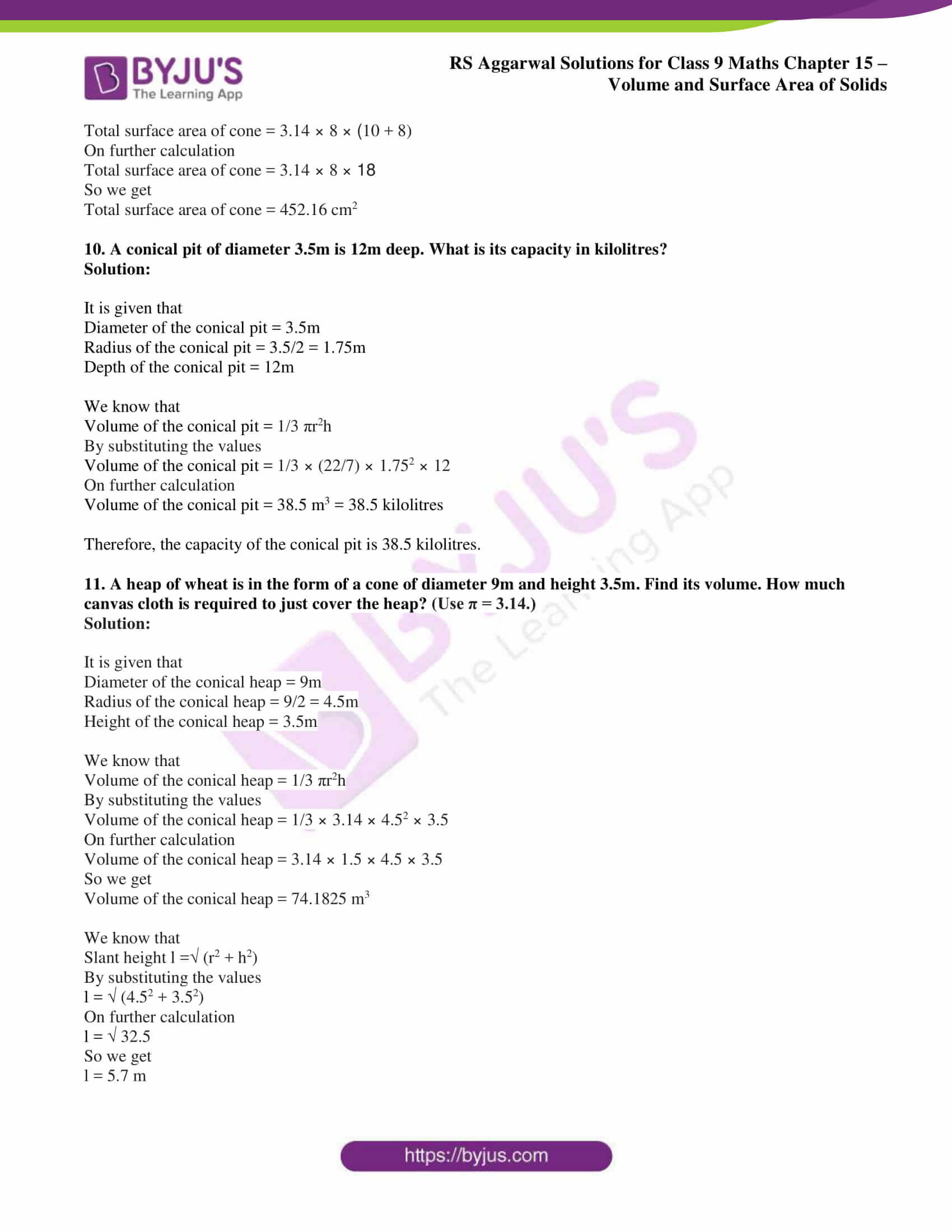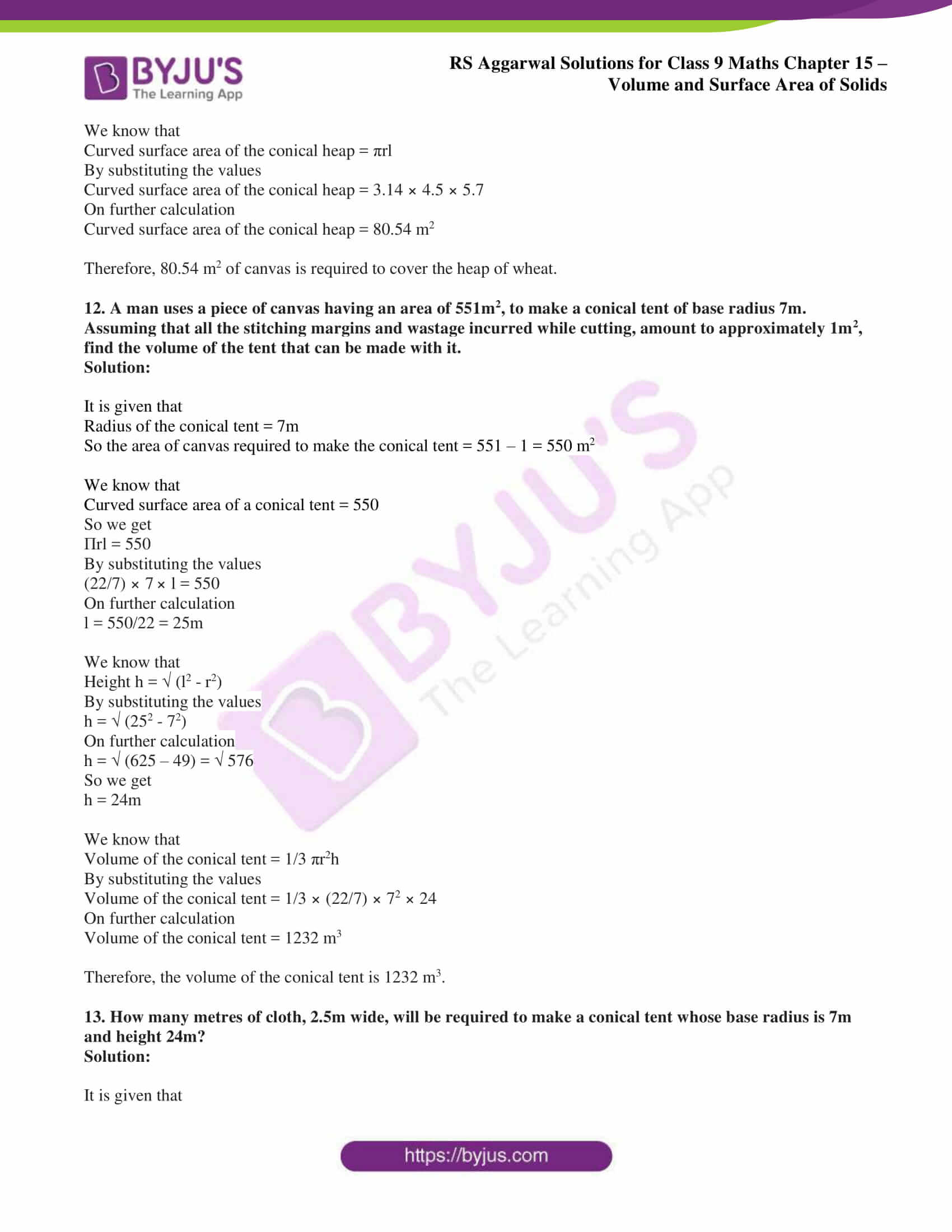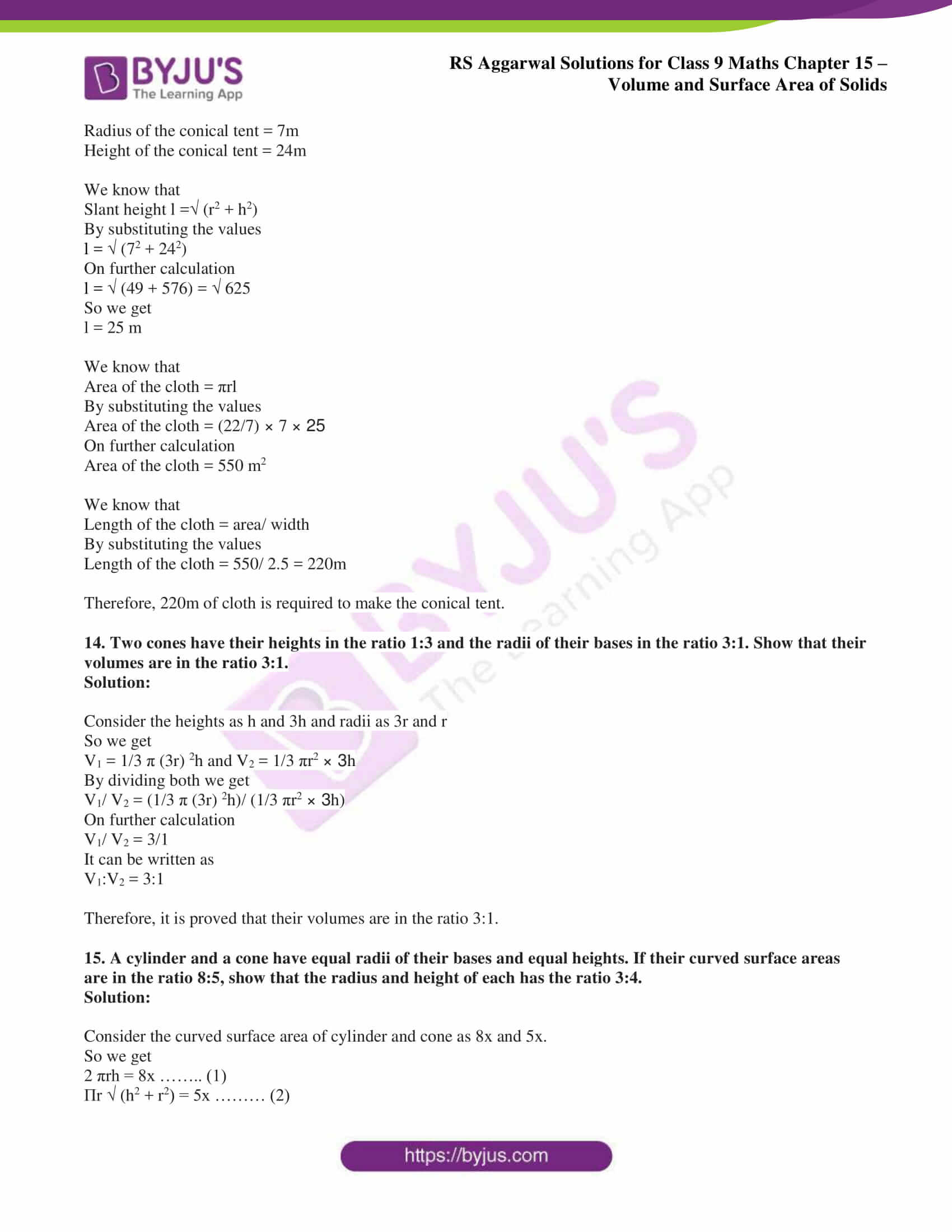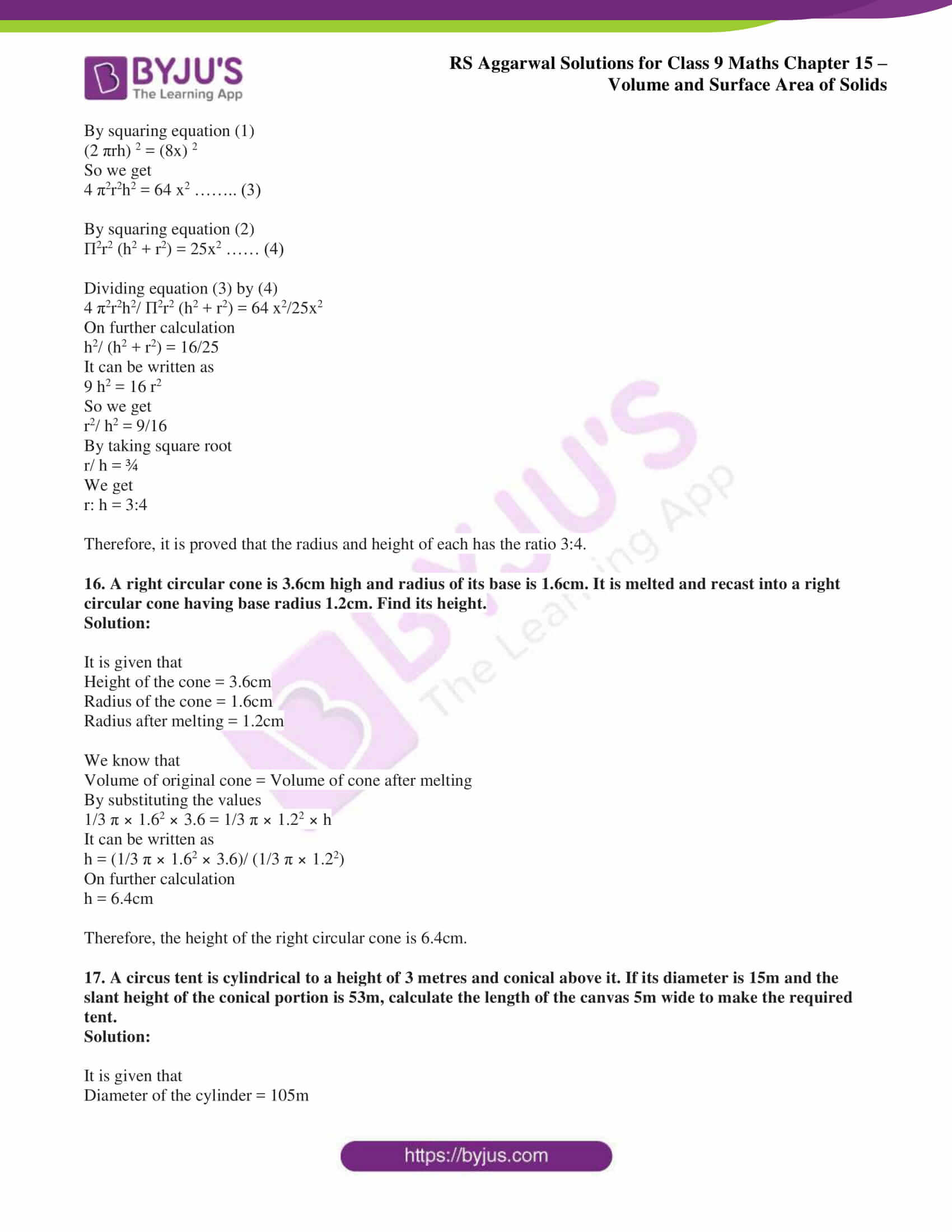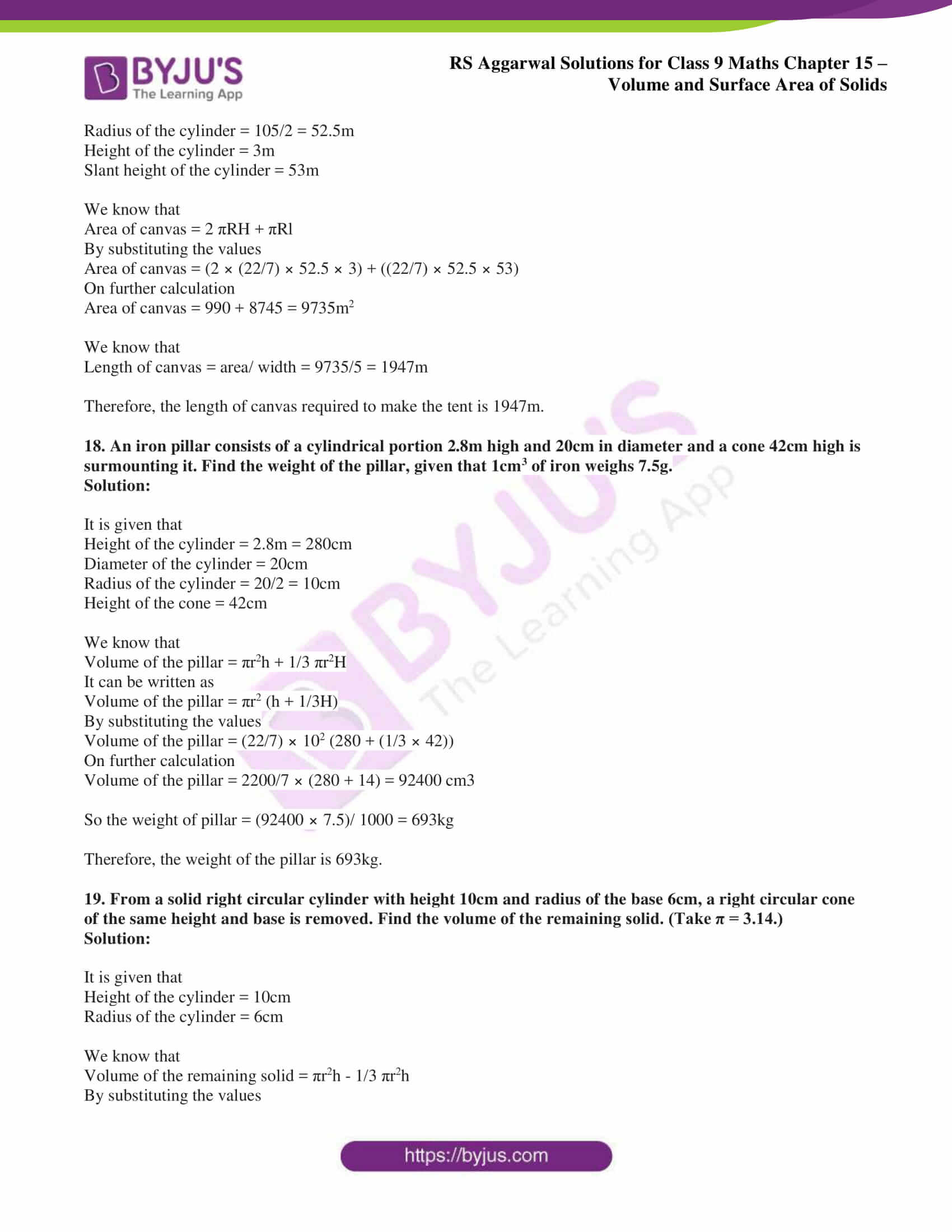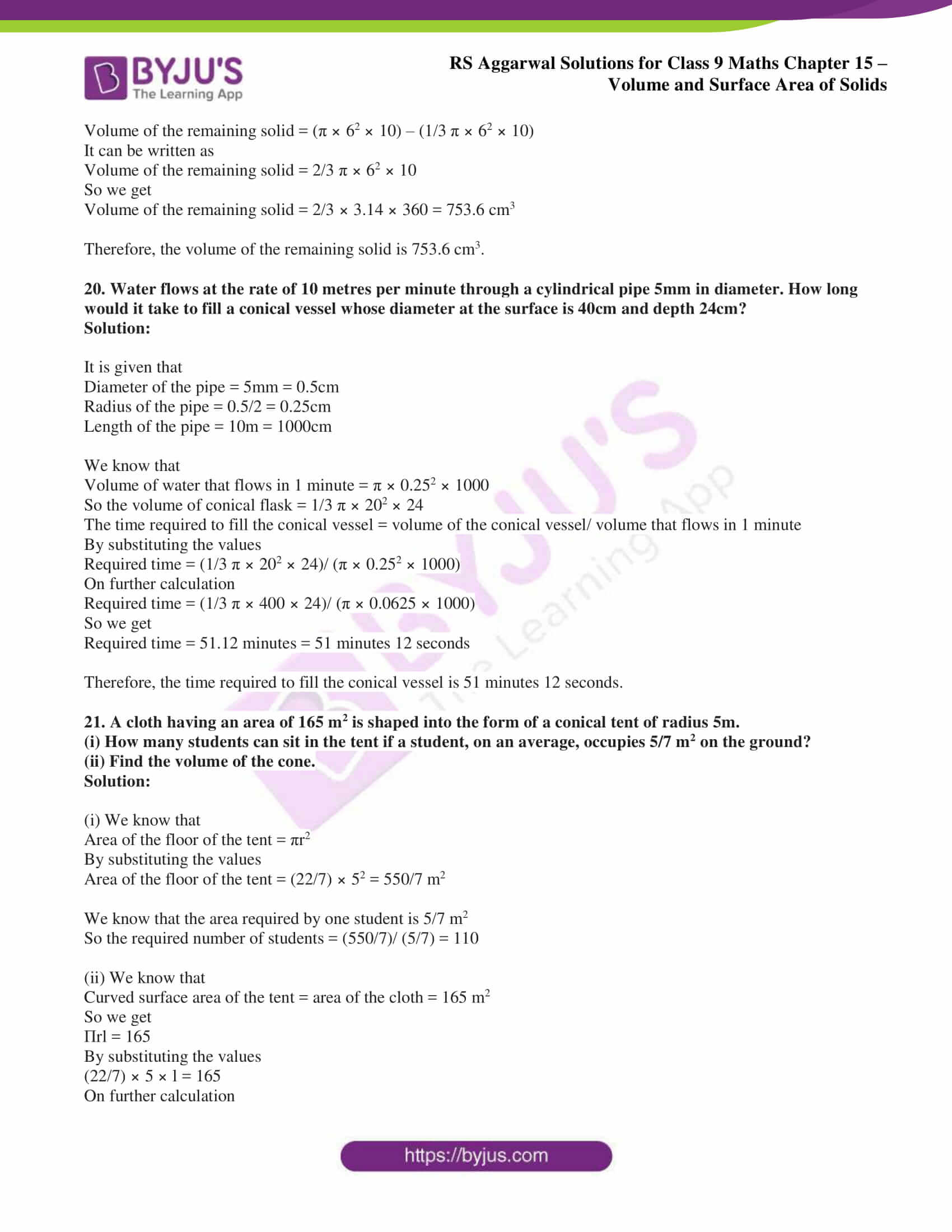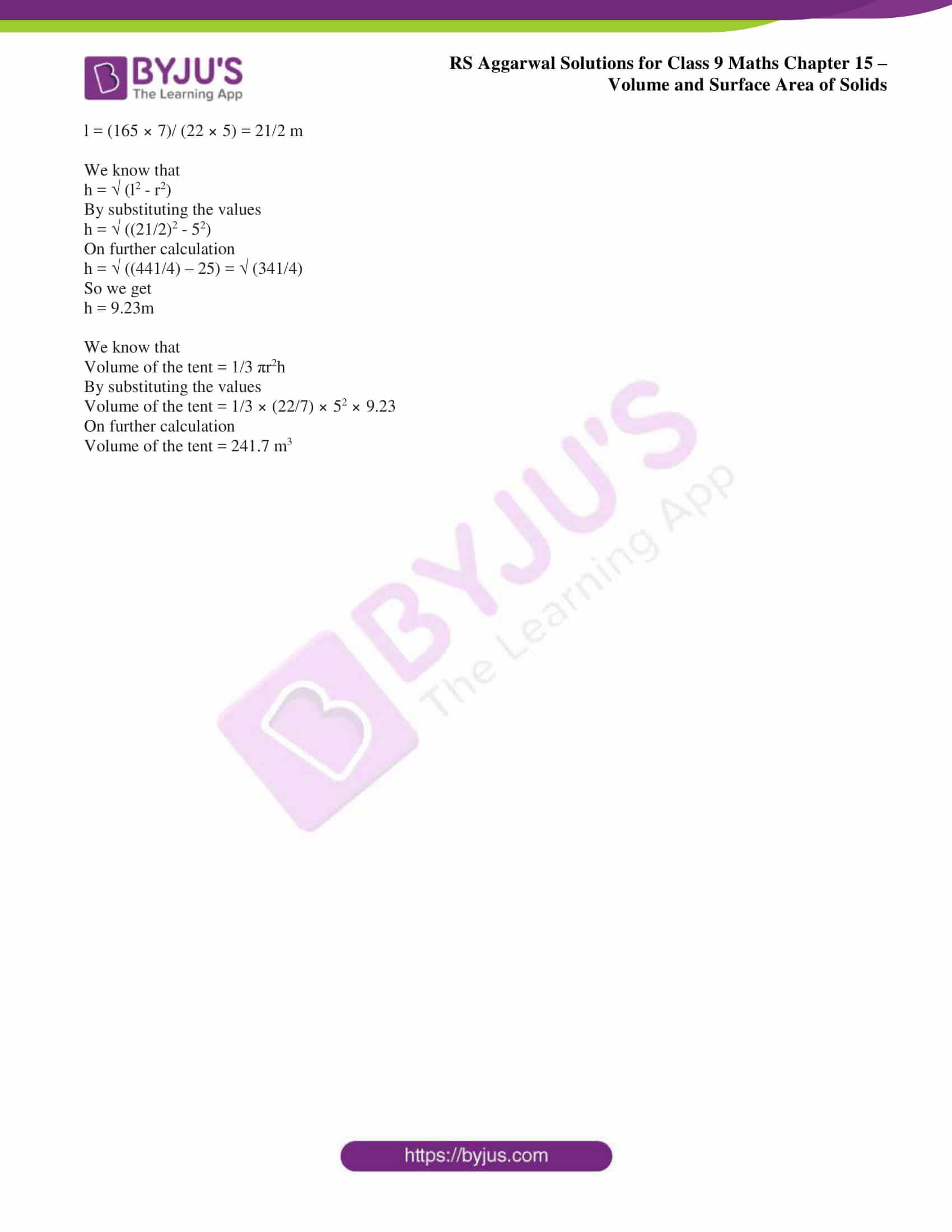## Access RS Aggarwal Solutions for Class 9 Chapter 15: Volumes and Surface Area of Solids Exercise 15C

1. Find the curved surface area of a cone with base radius 5.25cm and slant height 10cm.

Solution:

It is given that

Radius of the cone = 5.25cm

Slant height of the cone = 10cm

We know that

Curved surface area of the cone = πrl

By substituting the values

Curved surface area of the cone = (22/7) × 5.25 × 10

So we get

Curved surface area of the cone = 165 cm2

Therefore, the curved surface area of a cone is 165 cm2.

2. Find the total surface area of a cone, if its slant height is 21m and diameter of its base is 24m.

Solution:

It is given that

Diameter of the cone = 24m

Radius of the cone = 24/2 = 12m

Slant height of the cone = 21m

We know that

Total surface area of a cone = πr (l + r)

By substituting the values

Total surface area of a cone = (22/7) × 12 (21+12)

On further calculation

Total surface area of a cone = (22/7) × 12 × 33

So we get

Total surface area of a cone = 1244.57 m2

Therefore, the total surface area of a cone is 1244.57 m2.

3. A joker’s cap is in the form of a right circular cone of base radius 7cm and height 24cm. Find the area of the sheet required to make 10 such caps.

Solution:

It is given that

Radius of the cap = 7cm

Height of the cap = 24cm

We know that

Slant height of the cap

l = √ (r2 + h2)

By substituting the values

l = √ (72 + 242)

On further calculation

l = √ (49 + 576) = √ 625

So we get

l = 25cm

We know that

Curved surface area of one cap = πrl

By substituting the values

Curved surface area of one cap = (22/7) × 7 × 25

So we get

Curved surface area of one cap = 550 cm2

So the curved surface area of 10 conical caps = 10 × 550 = 5500 cm2

Therefore, the area of the sheet required to make 10 such caps is 5500 cm2.

4. The curved surface area of a cone is 308cm2 and its slant height is 14cm. Find the radius of the base and total surface area of the cone.

Solution:

Consider r as the radius of the cone

It is given that

Slant height of the cone = 14cm

Curved surface area of the cone = 308cm2

It can be written as

Πrl = 308

By substituting the values

(22/7) × r × 14 = 308

On further calculation

22 × r × 2 = 308

So we get

r = 308/ (22 × 2)

r = 7cm

We know that

Total surface area of a cone = Πr (l + r)

By substituting the values

Total surface area of a cone = (22/7) × 7 × (14 + 7)

On further calculation

Total surface area of a cone = 22 ×21 = 462 cm2

Therefore, the base radius of the cone is 7cm and the total surface area is 462 cm2.

5. The slant height and base diameter of a conical tomb are 25m and 14m respectively. Find the cost of whitewashing its curved surface at the rate of ₹ 12 per m2.

Solution:

It is given that

Radius of the cone = 7m

Slant height of the cone = 25m

We know that

Curved surface area of the cone = πrl

By substituting the values

Curved surface area of the cone = (22/7) × 7 × 25

So we get

Curved surface area of the cone = 550 m2

It is given that the cost of whitewashing = ₹ 12 per m2

So the cost of whitewashing 550 m2 area = ₹ 12 × 550 = ₹ 6600

Therefore, the cost of whitewashing its curved surface area is ₹ 6600.

6. A conical tent is 10m high and the radius of its base is 24m. Find the slant height of the tent. If the cost of 1m2 canvas is ₹ 70, find the cost of canvas required to make the tent.

Solution:

It is given that

Radius of the conical tent = 24m

Height of conical tent = 10m

We know that

Slant height of conical tent can be written as

l = √ (r2 + h2)

By substituting the values

l = √ (242 + 102)

On further calculation

l = √ (576 + 100) = √ 676

So we get

l = 26m

We know that

Curved surface area of conical tent = πrl

By substituting the values

Curved surface area of conical tent = (22/7) × 24 × 26

So we get

Curved surface area of conical tent = (13728/7) m2

It is given that the cost of 1m2 canvas = ₹ 70

So the cost of (13728/7) m2 canvas = ₹ 70 × (13728/7) = ₹ 137280

Therefore, the slant height of the tent is 26m and the cost of canvas required to make the tent is ₹ 137280.

7. A bus stop is barricaded from the remaining part of the road by using 50 hollow cones made of recycled cardboard. Each one has a base diameter of 40cm and height 1m. If the outer side of each of the cones is to be painted and the cost of painting is ₹ 25 per m2, what will be the cost of painting all these cones? (Use π = 3.14 and √1.04 = 1.02.)

Solution:

It is given that

Radius of the cone = 20cm = 0.2m

Height of the cone = 1m

We know that

Slant height l = √ (r2 + h2)

By substituting the values

l = √ (0.22 + 12)

On further calculation

l = √ (0.04 + 1) = √ 1.04

So we get

l = 1.02m

We know that

Curved surface area of cone = πrl

By substituting the values

Curved surface area of cone = 3.14 × 0.2 × 1.02

On further calculation

Curved surface area of cone = 0.64056 m2

So the curved surface area of 50 cones = 50 × 0.64056 = 32.028 m2

It is given that

Cost of painting = ₹ 25 per m2

So the cost of painting 32.028 m2 area = ₹ 25 × 32.028 = ₹ 800.70

Therefore, the cost of painting all these cones is ₹ 800.70.

8. Find the volume, curved surface area and the total surface area of a cone having base radius 35cm and height 12cm.

Solution:

It is given that

Radius of the cone = 35cm

Height of the cone = 12cm

We know that

Volume of the cone = 1/3 πr2h

By substituting the values

Volume of the cone = 1/3 × (22/7) × 352 × 12

On further calculation

Volume of the cone = 15400 cm3

We know that

Slant height l = √ (r2 + h2)

By substituting the values

l = √ (352 + 122)

On further calculation

l = √ 1369

So we get

l = 37 cm

We know that

Curved surface area of a cone = πrl

By substituting the values

Curved surface area of a cone = (22/7) × 35 × 37

So we get

Curved surface area of a cone = 4070 cm2

We know that

Total surface area of cone = πr (l + r)

By substituting the values

Total surface area of cone = (22/7) × 35 × (37 + 35)

On further calculation

Total surface area of cone = 22 × 5 × 72

So we get

Total surface area of cone = 7920 cm2

9. Find the volume, curved surface area and the total surface area of a cone whose height is 6cm and slant height 100cm. (Take π = 3.14.)

Solution:

It is given that

Height of the cone = 6cm

Slant height of the cone b= 10cm

We know that

Radius of the cone = √ (l2 – h2)

By substituting the values

Radius of the cone = √ (102 – 62)

On further calculation

Radius of the cone = √ (100 – 36) = √ 64

So we get

Radius of the cone = 8cm

We know that

Volume of the cone = 1/3 πr2h

By substituting the values

Volume of the cone = 1/3 × 3.14 × 82 × 6

On further calculation

Volume of the cone = 401.92 cm3

We know that

Curved surface area of a cone = πrl

By substituting the values

Curved surface area of a cone = 3.14 × 8 × 10

So we get

Curved surface area of a cone = 251.2 cm2

We know that

Total surface area of cone = πr (l + r)

By substituting the values

Total surface area of cone = 3.14 × 8 × (10 + 8)

On further calculation

Total surface area of cone = 3.14 × 8 × 18

So we get

Total surface area of cone = 452.16 cm2

10. A conical pit of diameter 3.5m is 12m deep. What is its capacity in kilolitres?

Solution:

It is given that

Diameter of the conical pit = 3.5m

Radius of the conical pit = 3.5/2 = 1.75m

Depth of the conical pit = 12m

We know that

Volume of the conical pit = 1/3 πr2h

By substituting the values

Volume of the conical pit = 1/3 × (22/7) × 1.752 × 12

On further calculation

Volume of the conical pit = 38.5 m3 = 38.5 kilolitres

Therefore, the capacity of the conical pit is 38.5 kilolitres.

11. A heap of wheat is in the form of a cone of diameter 9m and height 3.5m. Find its volume. How much canvas cloth is required to just cover the heap? (Use π = 3.14.)

Solution:

It is given that

Diameter of the conical heap = 9m

Radius of the conical heap = 9/2 = 4.5m

Height of the conical heap = 3.5m

We know that

Volume of the conical heap = 1/3 πr2h

By substituting the values

Volume of the conical heap = 1/3 × 3.14 × 4.52 × 3.5

On further calculation

Volume of the conical heap = 3.14 × 1.5 × 4.5 × 3.5

So we get

Volume of the conical heap = 74.1825 m3

We know that

Slant height l =√ (r2 + h2)

By substituting the values

l = √ (4.52 + 3.52)

On further calculation

l = √ 32.5

So we get

l = 5.7 m

We know that

Curved surface area of the conical heap = πrl

By substituting the values

Curved surface area of the conical heap = 3.14 × 4.5 × 5.7

On further calculation

Curved surface area of the conical heap = 80.54 m2

Therefore, 80.54 m2 of canvas is required to cover the heap of wheat.

12. A man uses a piece of canvas having an area of 551m2, to make a conical tent of base radius 7m. Assuming that all the stitching margins and wastage incurred while cutting, amount to approximately 1m2, find the volume of the tent that can be made with it.

Solution:

It is given that

Radius of the conical tent = 7m

So the area of canvas required to make the conical tent = 551 – 1 = 550 m2

We know that

Curved surface area of a conical tent = 550

So we get

Πrl = 550

By substituting the values

(22/7) × 7 × l = 550

On further calculation

l = 550/22 = 25m

We know that

Height h = √ (l2 – r2)

By substituting the values

h = √ (252 – 72)

On further calculation

h = √ (625 – 49) = √ 576

So we get

h = 24m

We know that

Volume of the conical tent = 1/3 πr2h

By substituting the values

Volume of the conical tent = 1/3 × (22/7) × 72 × 24

On further calculation

Volume of the conical tent = 1232 m3

Therefore, the volume of the conical tent is 1232 m3.

13. How many metres of cloth, 2.5m wide, will be required to make a conical tent whose base radius is 7m and height 24m?

Solution:

It is given that

Radius of the conical tent = 7m

Height of the conical tent = 24m

We know that

Slant height l =√ (r2 + h2)

By substituting the values

l = √ (72 + 242)

On further calculation

l = √ (49 + 576) = √ 625

So we get

l = 25 m

We know that

Area of the cloth = πrl

By substituting the values

Area of the cloth = (22/7) × 7 × 25

On further calculation

Area of the cloth = 550 m2

We know that

Length of the cloth = area/ width

By substituting the values

Length of the cloth = 550/ 2.5 = 220m

Therefore, 220m of cloth is required to make the conical tent.

14. Two cones have their heights in the ratio 1:3 and the radii of their bases in the ratio 3:1. Show that their volumes are in the ratio 3:1.

Solution:

Consider the heights as h and 3h and radii as 3r and r

So we get

V1 = 1/3 π (3r) 2h and V2 = 1/3 πr2 × 3h

By dividing both we get

V1/ V2 = (1/3 π (3r) 2h)/ (1/3 πr2 × 3h)

On further calculation

V1/ V2 = 3/1

It can be written as

V1:V2 = 3:1

Therefore, it is proved that their volumes are in the ratio 3:1.

15. A cylinder and a cone have equal radii of their bases and equal heights. If their curved surface areas are in the ratio 8:5, show that the radius and height of each has the ratio 3:4.

Solution:

Consider the curved surface area of cylinder and cone as 8x and 5x.

So we get

2 πrh = 8x …….. (1)

Πr √ (h2 + r2) = 5x ……… (2)

By squaring equation (1)

(2 πrh) 2 = (8x) 2

So we get

4 π2r2h2 = 64 x2 …….. (3)

By squaring equation (2)

Π2r2 (h2 + r2) = 25x2 …… (4)

Dividing equation (3) by (4)

4 π2r2h2/ Π2r2 (h2 + r2) = 64 x2/25x2

On further calculation

h2/ (h2 + r2) = 16/25

It can be written as

9 h2 = 16 r2

So we get

r2/ h2 = 9/16

By taking square root

r/ h = ¾

We get

r: h = 3:4

Therefore, it is proved that the radius and height of each has the ratio 3:4.

16. A right circular cone is 3.6cm high and radius of its base is 1.6cm. It is melted and recast into a right circular cone having base radius 1.2cm. Find its height.

Solution:

It is given that

Height of the cone = 3.6cm

Radius of the cone = 1.6cm

We know that

Volume of original cone = Volume of cone after melting

By substituting the values

1/3 π × 1.62 × 3.6 = 1/3 π × 1.22 × h

It can be written as

h = (1/3 π × 1.62 × 3.6)/ (1/3 π × 1.22)

On further calculation

h = 6.4cm

Therefore, the height of the right circular cone is 6.4cm.

17. A circus tent is cylindrical to a height of 3 metres and conical above it. If its diameter is 15m and the slant height of the conical portion is 53m, calculate the length of the canvas 5m wide to make the required tent.

Solution:

It is given that

Diameter of the cylinder = 105m

Radius of the cylinder = 105/2 = 52.5m

Height of the cylinder = 3m

Slant height of the cylinder = 53m

We know that

Area of canvas = 2 πRH + πRl

By substituting the values

Area of canvas = (2 × (22/7) × 52.5 × 3) + ((22/7) × 52.5 × 53)

On further calculation

Area of canvas = 990 + 8745 = 9735m2

We know that

Length of canvas = area/ width = 9735/5 = 1947m

Therefore, the length of canvas required to make the tent is 1947m.

18. An iron pillar consists of a cylindrical portion 2.8m high and 20cm in diameter and a cone 42cm high is surmounting it. Find the weight of the pillar, given that 1cm3 of iron weighs 7.5g.

Solution:

It is given that

Height of the cylinder = 2.8m = 280cm

Diameter of the cylinder = 20cm

Radius of the cylinder = 20/2 = 10cm

Height of the cone = 42cm

We know that

Volume of the pillar = πr2h + 1/3 πr2H

It can be written as

Volume of the pillar = πr2 (h + 1/3H)

By substituting the values

Volume of the pillar = (22/7) × 102 (280 + (1/3 × 42))

On further calculation

Volume of the pillar = 2200/7 × (280 + 14) = 92400 cm3

So the weight of pillar = (92400 × 7.5)/ 1000 = 693kg

Therefore, the weight of the pillar is 693kg.

19. From a solid right circular cylinder with height 10cm and radius of the base 6cm, a right circular cone of the same height and base is removed. Find the volume of the remaining solid. (Take π = 3.14.)

Solution:

It is given that

Height of the cylinder = 10cm

Radius of the cylinder = 6cm

We know that

Volume of the remaining solid = πr2h – 1/3 πr2h

By substituting the values

Volume of the remaining solid = (π × 62 × 10) – (1/3 π × 62 × 10)

It can be written as

Volume of the remaining solid = 2/3 π × 62 × 10

So we get

Volume of the remaining solid = 2/3 × 3.14 × 360 = 753.6 cm3

Therefore, the volume of the remaining solid is 753.6 cm3.

20. Water flows at the rate of 10 metres per minute through a cylindrical pipe 5mm in diameter. How long would it take to fill a conical vessel whose diameter at the surface is 40cm and depth 24cm?

Solution:

It is given that

Diameter of the pipe = 5mm = 0.5cm

Radius of the pipe = 0.5/2 = 0.25cm

Length of the pipe = 10m = 1000cm

We know that

Volume of water that flows in 1 minute = π × 0.252 × 1000

So the volume of conical flask = 1/3 π × 202 × 24

The time required to fill the conical vessel = volume of the conical vessel/ volume that flows in 1 minute

By substituting the values

Required time = (1/3 π × 202 × 24)/ (π × 0.252 × 1000)

On further calculation

Required time = (1/3 π × 400 × 24)/ (π × 0.0625 × 1000)

So we get

Required time = 51.12 minutes = 51 minutes 12 seconds

Therefore, the time required to fill the conical vessel is 51 minutes 12 seconds.

21. A cloth having an area of 165 m2 is shaped into the form of a conical tent of radius 5m.

(i) How many students can sit in the tent if a student, on an average, occupies 5/7 m2 on the ground?

(ii) Find the volume of the cone.

Solution:

(i) We know that

Area of the floor of the tent = πr2

By substituting the values

Area of the floor of the tent = (22/7) × 52 = 550/7 m2

We know that the area required by one student is 5/7 m2

So the required number of students = (550/7)/ (5/7) = 110

(ii) We know that

Curved surface area of the tent = area of the cloth = 165 m2

So we get

Πrl = 165

By substituting the values

(22/7) × 5 × l = 165

On further calculation

l = (165 × 7)/ (22 × 5) = 21/2 m

We know that

h = √ (l2 – r2)

By substituting the values

h = √ ((21/2)2 – 52)

On further calculation

h = √ ((441/4) – 25) = √ (341/4)

So we get

h = 9.23m

We know that

Volume of the tent = 1/3 πr2h

By substituting the values

Volume of the tent = 1/3 × (22/7) × 52 × 9.23

On further calculation

Volume of the tent = 241.7 m3

### Access other exercise solutions of Class 9 Maths Chapter 15: Volume and Surface Area of Solids

Exercise 15A Solutions 28 Questions

Exercise 15B Solutions 33 Questions

Exercise 15D Solutions 28 Questions

### RS Aggarwal Solutions Class 9 Maths Chapter 15 – Volume and Surface Area of Solids Exercise 15C

RS Aggarwal Solutions Class 9 Maths Chapter 15 Volume and Surface Area of Solids Exercise 15C tells us about the concept of a right circular cone and formulas which can be used in finding areas.

### Key features of RS Aggarwal Solutions for Class 9 Maths Chapter 15: Volume and Surface Area of Solids Exercise 15C

• Self assessment about the concepts and areas of weaknesses is made easy among students.
• Solving a huge number of problems help students to answer the questions in the board exam more efficiently.
• The problems in the textbook are according to exam pattern and weightage based on the CBSE guidelines.
• Shortcuts and various methods used to solve problems help students remember formulas easily.